# Parameter space exploration with Latin Hypercube in R¶

*This tutorial assumes you have read the tutorial on numerical integration. and the tutorial on bifurcation analysis

## Software required¶

### R¶

In order to run the R codes in this notebook in your own computer, you need to install the following software:

• R, along with the packages from CRAN:
• deSolve, a library for solving differential equations
• rootsolve: root finding and equilibrium and steady-state analyses.
• pse, a library to run parameter space exploration of models
• ggplot2, a library for plotting
• reshape2, for manipulating data.frames

To install R, download it from its homepage (Windows or Mac): http://www.r-project.org/. On Linux, you can install it using your distribution's prefered way, e.g.:

• Debian/Ubuntu: sudo apt-get install r-base
• Fedora: sudo yum install R
• Arch: sudo pacman -S r

To install the packages, all you have to do is run the following in the R prompt

install.packages(c("deSolve", "rootSolve, ""ggplot2", "pse"))



In :
library(ggplot2)
library(pse)
library(deSolve)
library(rootSolve)

#### For jupyter notebook only
options(jupyter.plot_mimetypes = 'image/png')
####


The R code presented here and some additional examples are available at https://github.com/diogro/ode_examples (thanks, Diogro!).

## Exploring the parameter space with the Latin Hypercube¶

### First example: two competitors in a Lotka-Volterra System¶

#### 1. Create a R function that runs the model once¶

Create a function that gets the model parameters and time sequence and outputs the final size of populations, by numerical integration. If argument runsteady=TRUE the function uses the runsteady function of package rootSolve to run numerical integration till a stationary point (hopefully, please see help of the function). If runsteady=FALSE the function uses the ode function to do the integration.

In :
oneRun <- function(X0, Y0, a, b,  times, runsteady=TRUE){
## parameters: initial sizes and competition coefficients
parameters <- c(a = a, b = b)
state <- c(X = X0, Y = Y0)
## The function to be integrated
LV <- function(t, state, parameters){
with(as.list(c(state, parameters)), {
dX = X*(1 - X - a*Y)
dY = Y*(1 - Y - b*X)
list(c(dX, dY))
})
}
## Integrating: runsteady to run untill convergence (be carefull using that!)
## Or the usual integration with the function ode
out <- runsteady(y = state, times = times, func = LV, parms = parameters)
return(out$y) # runsteady returns only final states } else{ out <- ode(y = state, times = times, func = LV, parms = parameters) return(out[nrow(out),-1]) # indexing to get final state values } }  #### 2. Create a R function for running the model for each combination of parameters¶ Create a function with mapply that gets a matrix of parameter combinations (combinations are lines and parameters are columns) and returns the output of the function above for each parameter combination. Use argument MoreArgs to input the values for additional arguments that are not in the matrix of parameter (arguments runsteady and times in this case). In : modelRun <- function (my.pars) { return(mapply(oneRun, my.pars[,1], my.pars[,2], my.pars[,3], my.pars[,4], MoreArgs=list(times=c(0,Inf), runsteady=TRUE))) }  #### 3. Set the hypercube¶ Now prepare the hypercube. You have to specify: • A character vector to name the parameters. In this case the initial conditions and the two competition coefficients In : factors <- c("X0", "Y0", "a", "b")  • The probability quantile function used to draw values of each parameter. If you do not information on the distribution of parameters use uniform distribution to have equiprobable values in a range from min to max: In : q <- c("qunif", "qunif", "qunif", "qunif")  • A list of the parameters of the above probability distributions. For the uniform the parameters are minimum and maximun values. So in this case each element of the list is another listwith the max and min of the corresponding model parameter, in the same order as above In : q.arg <- list(list(min=0, max=1), list(min=0, max=1), list(min=0, max=2), list(min=0, max=2))  #### 4. Run the hypercube¶ Now create the hypercube and run the model for each combination of parameters with LHS, the working horse function of pse package. The argument N=200 sets 200 combinations of parameters, please see help for further information In : myLHS <- LHS(model=modelRun, factors=factors, N=200, q=q, q.arg=q.arg, nboot=100) # use nboot=0 if do not need partial correlations  #### 5. Explore your results¶ And now use exploratory statistics to identify interesting regions of the parameter space. The pse package provides some options like: ###### Cumulative distribution of outputs.¶ Shows that about 40% of the population sizes are$\simeq 0$, so coexistence is not granted: In : plotecdf(myLHS, stack=TRUE, xlab="Population relative size")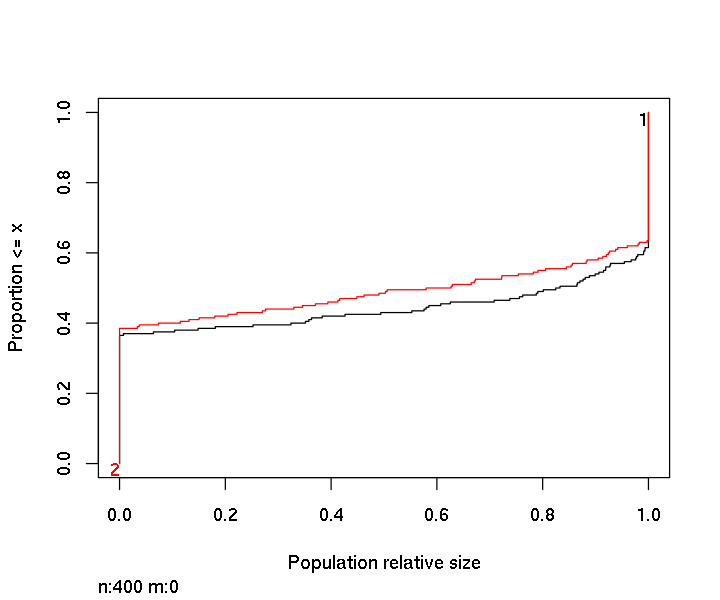##### Scatterplots of response x parameter values¶ Again we see that many runs resulted in exclusion of one of the species. We see also that initial conditions do not affect the final result, but competition coefficients do: In : plotscatter(myLHS, add.lm=FALSE)##### Partial correlation plots¶ Partial correlations are the non-parametric correlation of each output with each parameter, with the effects of the other variables partialed out. Confidence bars crossing the zero horizontal line indicate no-significant partial correlation. These plots show that final population sizes have a strong correlation with competition coefficients. In : plotprcc(myLHS)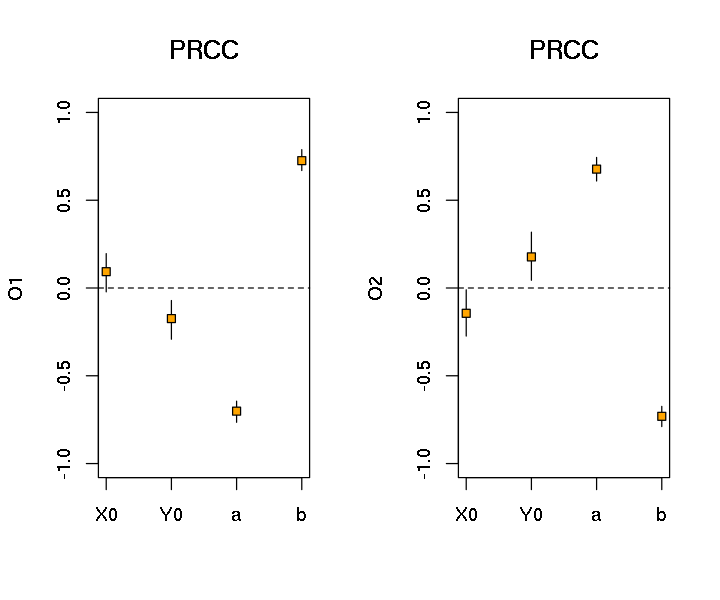##### Non-standard analyses¶ The package pse allows to extract a table with the outputs for each combination of parameters. In : ## get.data export a table with parameter values hypercube <- get.data(myLHS) ## get.results exports the outputs hypercube <- cbind(hypercube, get.results(myLHS)) # appending columns of outputs to the hypercube matrix names(hypercube)[5:6] <- c("X", "Y") # cosmetic  And now you are free to use any exploratory tool to identify interesting regions of the parameter space There is not a single recipe, but a first guess is to try univariate and then bivariate and multivariate analyses. In some case you can also use linear models such as multiple regressions to identify patterns. For instance, we already know that there is coexistence in this parameter space. But in how many runs? In : sum(hypercube$X>1e-6&hypercube$Y>1e-6)  Out: 52 Which parameter combinations ensue coexistence? Univariate exploration with boxplot shows that there is an upper bound of coefficients to have coexistence In : ## Create a logical variable to flag coexistence hypercube$coexistence <- hypercube$X>1e-6&hypercube$Y>1e-6
## boxplots
par(mfrow=c(2,2))
boxplot(X0~coexistence, data=hypercube, xlab="Coexistence", main="X0")
boxplot(Y0~coexistence, data=hypercube, xlab="Coexistence", main="Y0")
boxplot(a~coexistence, data=hypercube, xlab="Coexistence", main="a")
boxplot(b~coexistence, data=hypercube, xlab="Coexistence", main="b")
par(mfrow=c(1,1))Bivariate plots shows that coexistence is possible only if both coeficients are less than one, roughly (blue dots):

In :
plot(a~b, data=hypercube, type="n") ## to scale the plot for the whole parameter space
points(a~b, data=hypercube, subset=hypercube$X>1e-6&hypercube$Y>1e-6, col="blue")
points(a~b, data=hypercube, subset=!(hypercube$X>1e-6&hypercube$Y>1e-6), col="red")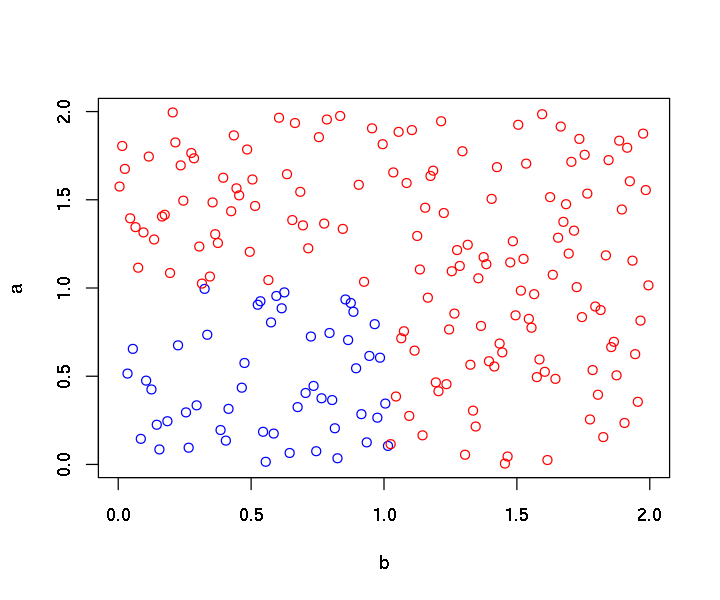Further exploration can reveal more!

### A more complicated example¶

Let's check a well-known result: in which conditions disease spreads in a Suscetible-Infected-Recovered epidemic model (SIR).

#### 1. R function for the model¶

In :
oneRun.sir <- function(S0, I0, R0, r, a, time=seq(0, 50, by = 0.01)){
## parameters: transmission and recovering rates
parameters <- c(r = r, a = a)
## initial population sizes: start with a single infected individual to test invasibility
state <- c(S = S0, I = I0, R = R0)
## The function to be integrated
sir <- function(t, state, parameters){
with(as.list(c(state, parameters)), {
dS = -r*S*I
dI = r*S*I - a*I
dR = a*I
list(c(dS, dI, dR))
})
}
## Integrating
out <- ode(y = state, times = time, func = sir, parms = parameters)
return(any(out[,3]>I0)) # returns a logical: any infected number larger than initial number?
}


#### 2. R function to run the model for each parameter combinations¶

As we are interested in disease spread we restrict I0 to one and R0 to zero.

In :
modelRun.sir <- function (my.pars) {
return(mapply(oneRun.sir, my.pars[,1], 1, 0, my.pars[,2], my.pars[,3]))
}


#### 3. Set the hypercube¶

In :
## A string vector to name the parameters
factors <- c("S0", "r", "a")
## The probability quantile function used to draw values of each parameter
q <- c("qunif", "qunif", "qunif")
## A list of the parameters of the above probability distributions
q.arg <- list(list(min=10, max=200), list(min=0.001, max=0.01), list(min=0, max=1))


#### 4. Run the hypercube¶

In :
myLHS <- LHS(model=modelRun.sir, factors=factors, N=200, q=q, q.arg=q.arg)


#### 5. Explore the results¶

Let's start looking at the effect of each parameter. with a boxplot of each parameter value in function of the occurrence of disease spread.

In :
##Table of parameter combinations with get.data
hypercube <- get.data(myLHS)
## adding the output for each combination with get.results
hypercube$Spread <- get.results(myLHS) ## box plots parameter ~ occurence of spread (logical) par(mfrow=c(1,3)) boxplot(S0~Spread, data=hypercube, xlab="Disease spread", ylab="Initial number of susceptibles") boxplot(r~Spread, data=hypercube, xlab="Disease spread", ylab="Transmission rate") boxplot(a~Spread, data=hypercube, xlab="Disease spread", ylab="Recovering rate") par(mfrow=c(1,1))You can also explore relatioships between parameters conditioned to the output. This is relationship of parameter$a$and$r$when spread occured or not. We'll restrict our exploration to populations with initial size < median of all initial size. In : plot(a~r, data=hypercube, type="n") # to show all ranges of the parameters points(a~r, data=hypercube, subset=S0<median(S0)&Spread==1, col="red") points(a~r, data=hypercube, subset=S0<median(S0)&Spread==0, col="blue")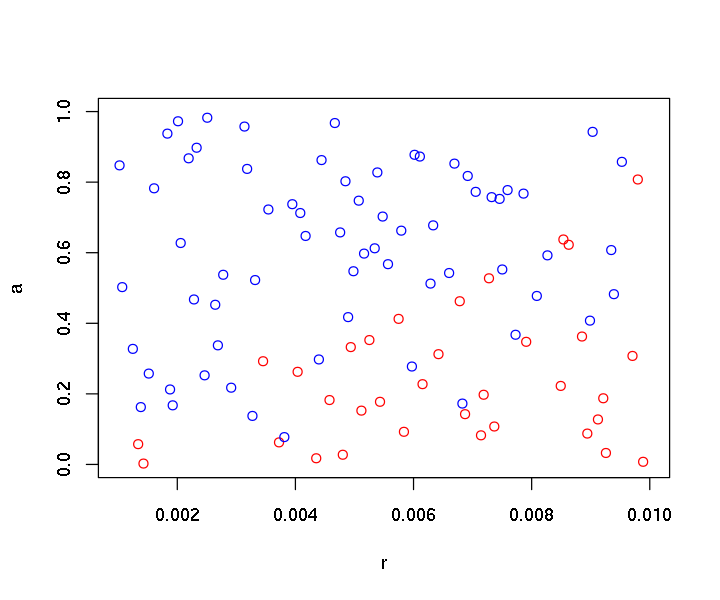A next step is to explore relationships between ratios of two parameters in function of additional parameters: In : plot(I(a/r)~S0, data=hypercube, type="n") # to show all ranges of the parameters points(I(a/r)~S0, subset=Spread==1, data=hypercube, col="red") points(I(a/r)~S0, subset=Spread==0, data=hypercube, col="blue") abline(0,1) ## equivalence line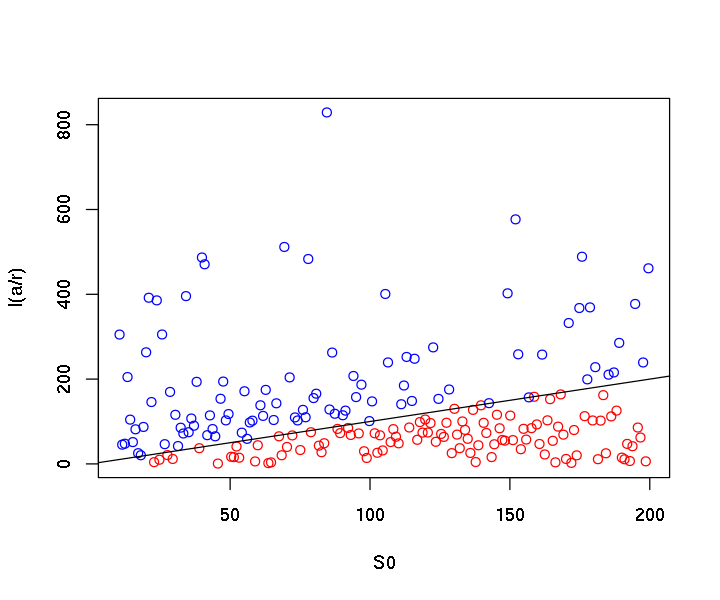BINGO! Disease spreads (red dots) if$S_0 > \frac{a}{r}\$